## Thursday, 7 August 2014

### chapter-21 Worked Out Examples

 Example: 19
 Show that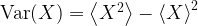${\rm{Var}}(X) = \left\langle {{X^2}} \right\rangle - {\left\langle X \right\rangle ^2}$
 Solution: 19${\rm{Var}}(X) = \left\langle {{{\left( {X - \left\langle X \right\rangle } \right)}^2}} \right\rangle \;$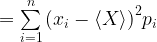$= \sum\limits_{i = 1}^n {{{\left( {{x_i} - \left\langle X \right\rangle } \right)}^2}{p_i}}$ {where the symbols have their usual meaning}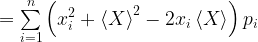$= \sum\limits_{i = 1}^n {\left( {x_i^2 + {{\left\langle X \right\rangle }^2} - 2{x_i}\left\langle X \right\rangle } \right){p_i}}$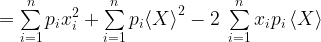$= \sum\limits_{i = 1}^n {{p_i}x_i^2} + \sum\limits_{i = 1}^n {{p_i}{{\left\langle X \right\rangle }^2}} - 2\;\sum\limits_{i = 1}^n {{x_i}{p_i}\left\langle X \right\rangle }$
Since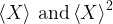$\left\langle X \right\rangle \,{\rm{and}}\,{\left\langle X \right\rangle ^2}$ are constants, they can be taken out of the summation in the second and third terms. Also, note that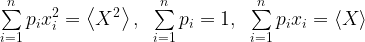$\sum\limits_{i = 1}^n {{p_i}x_i^2} = \left\langle {{X^2}} \right\rangle ,\;\;\sum\limits_{i = 1}^n {{p_i} = 1} ,\;\;\sum\limits_{i = 1}^n {{p_i}{x_i}} = \left\langle X \right\rangle \;$
so that,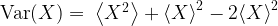${\rm{Var}}(X) = \;\left\langle {{X^2}} \right\rangle + {\left\langle X \right\rangle ^2} - 2{\left\langle X \right\rangle ^2}$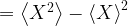$= \left\langle {{X^2}} \right\rangle - {\left\langle X \right\rangle ^2}$
which proves the assertion.
The relation is important and useful since it gives us the variance directly in terms of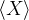$\left\langle X \right\rangle$ and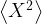$\left\langle {{X^2}} \right\rangle$. You are urged to try using this relation to calculate variance in the examples of variance we’ve discussed earlier.
 Example: 20
 Two cards are drawn simultaneously from a well-shuffled deck of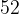$52$ cards. Find the mean and variance of the number of kings.
 Solution: 20
The number of kings is the random variable here. Call it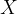$X$. The values that$X$ can take are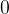$0$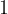$1$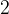$2$. The probabilities of the various values are easily calculated: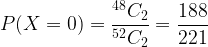$P(X = 0) = \dfrac{{^{48}{C_2}}}{{^{52}{C_2}}} = \dfrac{{188}}{{221}}$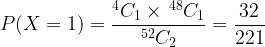$P(X = 1) = \dfrac{{^4{C_1} \times {\,^{48}}{C_1}}}{{^{52}{C_2}}} = \dfrac{{32}}{{221}}$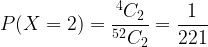$P(X = 2) = \dfrac{{^4{C_2}}}{{^{52}{C_2}}} = \dfrac{1}{{221}}$
The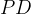$PD$ of$X$ is thereforeThe mean is simply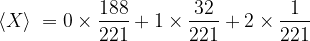$\left\langle X \right\rangle \; = 0 \times \dfrac{{188}}{{221}} + 1 \times \dfrac{{32}}{{221}} + 2 \times \dfrac{1}{{221}}$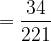$= \dfrac{{34}}{{221}}$
To calculate the variance, we first calculate$\left\langle {{X^2}} \right\rangle$: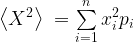$\left\langle {{X^2}} \right\rangle \; = \sum\limits_{i = 1}^n {x_i^2} {p_i}$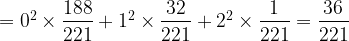$= {0^2} \times \dfrac{{188}}{{221}} + {1^2} \times \dfrac{{32}}{{221}} + {2^2} \times \dfrac{1}{{221}} = \dfrac{{36}}{{221}}$
Thus, the variance is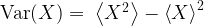${\rm{Var}}(X) = \;\left\langle {{X^2}} \right\rangle - {\left\langle X \right\rangle ^2}$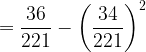$= \dfrac{{36}}{{221}} - {\left( {\dfrac{{34}}{{221}}} \right)^2}$$= \dfrac{{6800}}{{48841}}$